Prime Zeta Function

The prime zeta function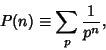(1)

where the sum is taken over Primes is a generalization of the Riemann Zeta Function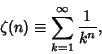(2)

where the sum is over all integers. The prime zeta function can be expressed in terms of the Riemann Zeta Function by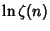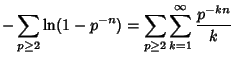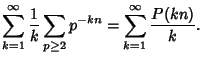(3)

Inverting then gives(4)

where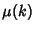is the Möbius Function. The values for the first few integers starting with two are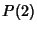(5)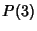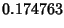(6)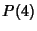(7)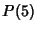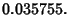(8)